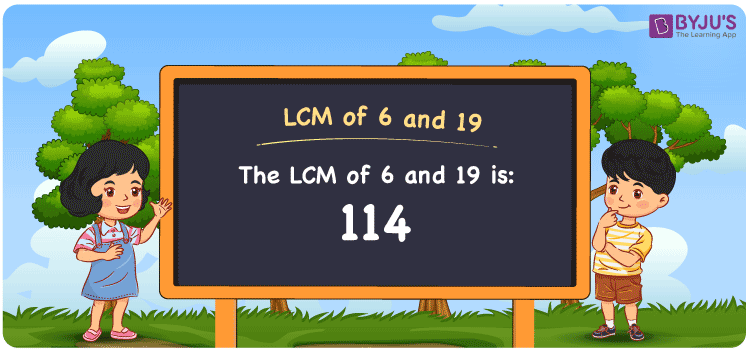# LCM of 6 and 19

LCM of 6 and 19 is 114. The value evenly divisible by 6 and 19 is the LCM value. The multiples which are common among 6 and 19 will help you to get the least common multiple of 6 and 19. The multiples of 6 are (6, 12, 18, 24, 30, 36, 42, 48, 54, 60, ….) and the multiples of 19 are (19, 38, 57, 76, 95, 114, 133, 152, 171, 190, ….) respectively. Students can find the LCM of the numbers using methods like the prime factorisation, division and by listing out the multiples.

Also read: Least common multiple

## What is LCM of 6 and 19?

The answer to this question is 114. The LCM of 6 and 19 using various methods is shown in this article for your reference. The LCM of two non-zero integers, 6 and 19, is the smallest positive integer 114 which is divisible by both 6 and 19 with no remainder.## How to Find LCM of 6 and 19?

LCM of 6 and 19 can be found using three methods:

• Prime Factorisation
• Division method
• Listing the multiples

### LCM of 6 and 19 Using Prime Factorisation Method

The prime factorisation of 6 and 19, respectively, is given by:

6 = 2 x 3 = 2¹ x 3¹

19 = 19¹

LCM (6, 19) = 114

### LCM of 6 and 19 Using Division Method

We’ll divide the numbers (6, 19) by their prime factors to get the LCM of 6 and 19 using the division method (preferably common). The LCM of 6 and 19 is calculated by multiplying these divisors.

 2 6 19 3 3 19 19 1 19 x 1 1

No further division can be done.

Hence, LCM (6, 19) = 114

### LCM of 6 and 19 Using Listing the Multiples

To calculate the LCM of 6 and 19 by listing out the common multiples, list the multiples as shown below.

Multiples of 6 = 6, 12, 18, 24, 30, 36, 42, 48, 54, 60, . . . ., 102, 108, 114, . . . .

Multiples of 19 = 19, 38, 57, 76, 95, 114, 133, 152, 171, 190, . . . ., 76, 95, 114, . . . .

LCM (6, 19) = 114

## Video Lesson on Applications of LCM## LCM of 6 and 19 Solved Examples

Question: The product of two numbers is 114. Find the LCM if GCD is 1.

Solution:

Given

Product = 114

GCD = 1

LCM x GCD = Product

LCM = Product/GCD

LCM = 114/1

LCM = 114

Therefore, the LCM of 6 and 19 is 114.

## Frequently Asked Questions on LCM of 6 and 19

### Determine the GCF if the LCM of 6 and 19 is 114.

We know that

LCM x GCF = 6 x 19

As LCM (6, 19) is 114

114 x GCF = 114

GCF = 114/114 = 1

### What is the LCM of 6 and 19?

The LCM of 6 and 19 is 114.

### Write the relation between GCF and LCM of 6 and 19.

The relation between GCF and LCM of 6 and 19 is

LCM x GCF = 6 x 19

LCM x GCF = 114

### The LCM and GCD of two numbers are 114 and 1. If one number is 19, find the other.

Consider b as the other number

GCD x LCM = 19 x b

b = (1 x 114)/19

b = 6

Hence, the other number is 6.

### In the numbers 50, 40, 28, 114, find the LCM of 6 and 19.

The LCM of 6 and 19 is the smallest common multiple divisible evenly by the given numbers. 114 is the number which satisfies this condition.